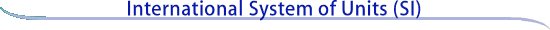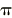Units outside the SI

Certain units are not part of the International System of Units, that is, they are outside the SI, but are important and widely used. Consistent with the recommendations of the International Committee for Weights and Measures (CIPM, Comité International des Poids et Mesures), the units in this category that are accepted for use with the SI are given in Table 6.

 Table 6.  Units outside the SI that are accepted for use with the SI Name Symbol Value in SI units minute (time) min 1 min = 60 s hour h 1 h = 60 min = 3600 s day d 1 d = 24 h = 86 400 s degree (angle) ° 1° = (/180) rad minute (angle)1= (1/60)° = (/10 800) rad second (angle)1= (1/60)= (/648 000) rad liter L 1 L = 1 dm3 = 10-3 m3 metric ton (a) t 1 t = 103 kg neper Np 1 Np = 1 bel (b) B 1 B = (1/2) ln 10 Np (c) electronvolt (d) eV 1 eV = 1.602 18 x 10-19 J, approximately unified atomic mass unit (e) u 1 u = 1.660 54 x 10-27 kg, approximately astronomical unit (f) au 1 au = 149 597 870 700 m, exactly (a) In many countries, this unit is called "tonne.'' (b) The bel is most commonly used with the SI prefix deci: 1 dB = 0.1 B. (c) Although the neper is coherent with SI units and is accepted by the CIPM, it has not been adopted by the General Conference on Weights and Measures (CGPM, Conférence Générale des Poids et Mesures) and is thus not an SI unit. (d) The electronvolt is the kinetic energy acquired by an electron passing through a potential difference of 1 V in vacuum. The value must be obtained by experiment, and is therefore not known exactly. (e) The unified atomic mass unit is equal to 1/12 of the mass of an unbound atom of the nuclide 12C, at rest and in its ground state. The value must be obtained by experiment, and is therefore not known exactly. (f) The astronomical unit of length was redefined by the XXVIII General Assembly of the International Astronomical Union (Resolution B2, 2012).The liter in Table 6 deserves comment. This unit and its symbol l were adopted by the CIPM in 1879. The alternative symbol for the liter, L, was adopted by the CGPM in 1979 in order to avoid the risk of confusion between the letter l and the number 1. Thus, although both l and L are internationally accepted symbols for the liter, to avoid this risk the preferred symbol for use in the United States is L. Neither a lowercase script letter l nor an uppercase script letter L are approved symbols for the liter.

Other units outside the SI that are currently accepted for use with the SI by NIST are given in Table 7. These units, which are subject to future review, should be defined in relation to the SI in every document in which they are used; their continued use is not encouraged. The CIPM currently accepts the use of all of the units given in Table 7 with the SI except for the curie, roentgen, rad, and rem. Because of the continued wide use of these units in the United States, NIST still accepts their use with the SI.

 Table 7.  Other units outside the SI that are currently accepted for use with the SI, subject to further review Name Symbol Value in SI units nautical mile 1 nautical mile = 1852 m knot 1 nautical mile per hour = (1852/3600) m/s are a 1 a = 1 dam2 = 102 m2 hectare ha 1 ha = 1 hm2 = 104 m2 bar bar 1 bar = 0.1 MPa = 100 kPa = 1000 hPa = 105 Pa ångström Å 1 Å = 0.1 nm = 10-10 m barn b 1 b = 100 fm2 = 10-28 m2 curie Ci 1 Ci = 3.7 x 1010 Bq roentgen R 1 R = 2.58 x 10-4 C/kg rad rad 1 rad = 1 cGy = 10-2 Gy rem rem 1 rem = 1 cSv = 10-2 Sv

Continue to
Rules and style conventions